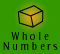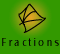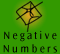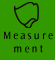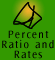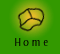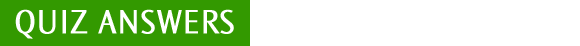| Meaning and models | Key ideas | Length and Perimeter |
| Area | Volume and Capacity | Mass |

 1 Kilo means 1000. There are 1000 m in 1 km 2 Micro means a millionth. 1 microsecond = one millionth of a second therefore, 1 000 000 microseconds = 1 second 3 (a) You would use length measure to measure the length of a table. Use metres for a large table. (b) You would use litres to measure volume. (c) You would use length measure to measure the length of a baby. Use centimetres. (d) You would use time units to measure the time to run 100 m. Use seconds. (e) You would use mass units to measure the mass of a large dog. Use kilograms. 4 Strictly speaking, only the second is an SI unit out of those given in the answers, therefore D. For more information read the meaning and models section. 5 Neither is heavier. 1 kg is 1 kg no matter what you are weighing.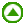1. 1400 metres = 1400 ÷ 1000 km = 1.4 kilometres (we are converting a smaller unit to a larger unit so we divide) 2. 2.637 kilograms = 2.637 x 1000 g = 2637 grams (we are converting a larger unit to a smaller unit so we multiply) 3. 4.5 kilolitres = 4.5 x 1000 litres = 4500 litres 4. Check your own answer
5.

Length of desk = 15.5 lots of 9

= 15.5 x 9

155 x 9 = 1395

so 15.5 x 9 = 139.5

Length of desk = 139.5 cm

6.

Matthew’s measurement appears to be more accurate as he has measured to the nearest hundredth of a centimetre, while Naomi has measured to the nearest tenth of a centimetre. However in most circumstances it would be unlikely that Matthew could accurately measure to the nearest hundredth of a centimetre - this is 10 times smaller than a millimetre.

7.
 (a) 385 centimetres = 385 ÷ 100 = 3.85 metres (b) 9.35 kilograms = 9.35 x 1000 = 9350 grams (c) 49 millilitres = 49 ÷ 1000 = 0.049 litres (d) 0.054 km = 0.054 x 1000 = 54 metres = 54 x 100 = 5400 centimetres (e) 8647 km = 8647 x 1000 = 8 647 000 metres
8.

Number of centimetres in 3 m 65 cm

= 300 cm + 65 cm

= 365 cm

9.

Length of Martin’s stride = 80 cm

Length of 60 strides = 60 x 80 = 4800 cm

Number of m = 4800 ÷ 100 = 48 m

10. How many 25 g in 1000 g?

1000 ÷ 25 = 40

Number of 25 g packets = 40

11. 9.00pm to 7.00am is 10 hours.

60 minutes in 1 hour

Number of minutes in 10 hours = 10 x 60 = 600

60 seconds in 1 minute

Number of minutes in 600 minutes = 600 x 60

= 36 000s

12. There are 1000 ml in a litre

So 0.325 l = 325 ml

Hence, 3250 ml is larger.1. a) 39 cm is 390 mm

b) 543 m = 543 x 100 cm = 54300 cm

c) 16790 mm = 16790 ÷ 10 (to get into cms) ÷ 100 (to get into m) = 16.790 m

d) 1.44 km = 1.44 x 1000 (to get into m) x 100 (to get into cm) x 10 (to get into mm) = 1.44 x 1 000 000 = 1440 000 mm

2. a) 340 mm = 34 cm

34 cm < 45 cm

b) 5009 m = 5.009 km

5.009 km < 12 km

c) 7000 mmm = 7 m

7 m < 8.5 m

d) 13 000 mm = 13 m

13 m < 1.3 km

3.Shape 1: Perimeter = 2.5 + 2 x 7 + 2 cm = 18.5 cm
Shape 2: Perimeter = 5 + 5 + 4.5 m = 14.5 m
Shape 3: Perimeter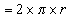= 2 x 3.14 x 2.7 = 16.96
NOTE that we have used 3.14 as an estimate for pi and that our answer has no units because we were given none in the question.

4.

We are told that the radius of a swimming pool is 1.5 metres. To find the perimeter of a circle, also called the circumference, we use the formula, circumference.

circumference of the pool = 2 x 3.14 x 1.5 = 9.42 metres

5.

I have estimated that my palm is about 10 cm wide, which is about right for a man.

The length of the table is 18 palm widths which is approximately 18 x 10 cm = 180 cm or 1.8 m
The width of the table is 12 palm widths which is approximately 12 x 10 cm = 120 cm or 1.2 m

When I actually measured my palm width I realised that it was only 8.5 cm wide. Therefore a more accurate measurement of the dimensions of the table would be:

The length of the table is 18 palm widths which is approximately 18 x 8.5 cm = 153 cm or 1.53 m
The width of the table is 12 palm widths which is approximately 12 x 8.5 cm = 102 cm or 1.02 m

These measurements are not exact as there would be some inaccuracy in using my palm as a tape measure.

6.

The length of a small suburban house block is 36.5 m and the width is 15.9 m.

The perimeter of a rectangular object can be found using the formula,

 Perimeter = 2 x length + 2 x width = 2 x 36.5 m + 2 x 15.9 m = 73 + 31.8 m = 104.80 m

Therefore the perimeter of the block is 104.80 m.

The new subdivided block will still be 36.5 m long but will only by 7.95 m (15.9 m divided by 2) wide.

 Perimeter = 2 x length + 2 x width = 2 x 36.5 m + 2 x 7.95 m = 73 + 15.9 m = 88.9 m

Therefore the perimeter of the new block is 88.9 m.

(It is worth noting that in an example such as this, the area of the new blocks will be half that of the original block, but the perimeter is not. Can you see why?)

1.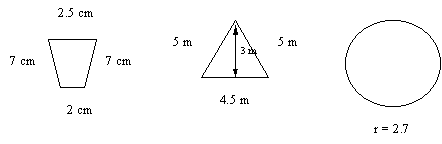The first shape is a trapezium. A = 1/2 x (a + b) x h, where 'a' and 'b' are the bases and 'h' is the perpendicular height.

 Area = 1/2 x (a + b) x h = 1/2 x (2 cm + 2.5 cm) x 7 cm = 1/2 x 4.5 cm x 7 cm = 15.75 cm2

The second shape is a triangle. A = 1/2 x b x h, 'b' is the base and 'h' refers to the perpendicular height.

 Area = 1/2 x b x h = 1/2 x 4.5 cm x 3 cm = 6.75 cm2

NOTE: The 'h' in the formula to calculate the area of a triangle ALWAYS refers to the perpendicular height.

The third shape is a circle. A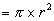where we have used 3.142 as an approximation for 'pi' and 'r' refers to the radius.

 Area= 3.142 x (2.7 cm)2 = 22.91 cm2 (rounded to 2 decimal places)

2.

The area of a circle is measured by. In this case we are told the radius is 4.5 cm

 Area= 3.142 x (4.5 cm2) = 3.142 x 20.25 cm2 = 63.63 cm2 (rounded to 2 decimal places)

3. The area of a parallelogram = base x perpendicular height

 Area = base x height = 12 cm x 3.4 cm = 40.8 cm2 (rounded to 2 decimal places)

4.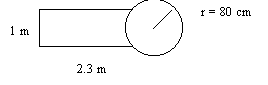To find the area of the bench we will first find the area of the circle and the the area of the rectangular portion.

We can see that both centimetres and metres have been used as units of measure. I will convert the centimetres to metres so that all measurements are in the same units and there is no room for error when we add the areas at the end.

80 cm = 0.8 m

Area of the circle:

 Area= 3.142 x (0.8 m2) = 3.142 x 0.64 m2 = 2.01 m2 (rounded to 2 decimal places)

Area of the rectangle:

 Area = length x width = 2.3 m x 1 m = 2.3 m2

Adding the two areas together, 2.01 m2 + 2.3 m2 = 4.31 m2.

So the approximate area of the bench is 4.31 m2. Note that this is only an approximation because we can see that one end of the rectangular bench has been overlapped by the circle.

5.

The tops of the desks are trapezium shaped. The formula to calculate the area of a trapezium is 1/2 x (a + b) x h where a and b are each side lengths.

As we have measurements in centimetres and metres we will convert all measurements to metres.

 Area = 1/2 x (b + a) x h = 1/2 x (1.1 m + 0.85 m) x 0.7 m = 0.68 m2 (rounded to 2 decimal places)

There are 12 desks so the total area of the tops of the desks if they are all jammed up together is 12 x 0.68 = 8.16 m2.

Therefore all 12 desks will not fit into a 8 m2 square room unless they are stacked on top of one another. 10 desks would fit into the room, taking up 6.8 m2 of space but there would still not be much extra room.

6.

Although the 3 parallelograms are of different sizes, they all have the same height of 1.8 m. (We note that the height does not have to be vertical, but it must be perpendicular to the base).

 Area1 = 1.8 m x 0.25 m = 0.45 m2 Area2 = 1.8 m x 0.48 m = 0.86 m2 Area3 = 1.8 m x 2.3 m = 4.14 m2

The total area that requires a non-slip coating is 5.45 m2.1.

The first solid is a cylinder. It has a uniform cross-section, so this means we can use the formula for the volume of a prism to its volume, V = A x h.

The base of the prism is a circle. The formula to find the area of a circle is, A. Therefore the formula to find the volume of the cylinder is, V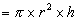Volume of a cylinder= 3.142 x 9 cm2 x 7.5 cm = 212.085 cm3

The second solid is a rectangular prism. Because a prism has a uniform cross-section we can find its volume by multiplying the area of its base by its height. V = A x h.

 Volume of a prism = A x h = 6 cm2 x 10 cm = 60 cm3
2.

This cube is also a rectangular prism. We are told the side length of its cubic base is 4 m. So, first we will find the area of its base.

To find the area of a square (the cubic base) we simply multiply its length by its length, which in this case is 4m. Therefore the area of the base is 16 m2.

To find the volume we apply the formula to find the volume of a prism.

 Volume of a prism = A x h = 16 m2 x 8 m = 128 m3
3.

This solid is also a prism, so we can use the same formula to find its volume.

 Volume of a prism = A x h = 9 cm2 x 5 cm = 45 cm31. a) There are 1000 kilograms in 1 tonne. b) 1 mL of water is equivalent to 1 cm3 of water. (See Volume and Capacity measurement facts) c) There are 1000 mg in 1 g. d) There are 1000 mg in 1g and there are 1000 g in 1 kg. So, there is 1000 x 1000 mg in 1 kg which is 1 000 000 mg. 2. a) car - use tonnes or kilograms b) book - use grams or kilograms (depending on how heavy the book feels) c) computer - use kilograms d) person - use kilograms e) cup of flour - grams f) feather - milligrams 3. See explanation at the beginning of this section. 4. a) 150 g flour, 100 g coconut, 200 g sugar, 300 g mixed fruit 150 + 100 + 200 + 300 = 750 g in total mass. b) 25 students weigh on average 45 kilograms. The students estimated total mass in kilograms is 25 x 45 kg = 1125 kg The students estimated total mass in tonnes is 1125 kg divided by 1000, which is 1.125 t.